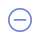Pokedex100 provides Pokemon coordinates all over 30 countries.

Found
{\$ hlist.name \$} 100 and {\$ hlist.length - 1 \$} more Pokémon with 100IV here click to show click to hide
 #{\$ x.number \$} {\$ x.name \$} {\$ x.iv \$} {\$ x.name \$} #{\$ x.number \$} {\$ x.name \$} {\$ x.iv \$} Upgrade to {\$ x.tier \$} to unlock
Data Is Only Accurate To Trainers Of Level 30 and Abovetotal: {\$ totalpokemons \$}
 #{\$ x.number \$} {\$ x.name \$}{\$ x.g == 1 ? "♂" : x.g == 2 ? "♀" : "" \$} {\$ x.iv >= 0 ? x.iv + "IV" : "" \$} {\$ x.cp > 0 ? x.cp + "CP" : "" \$} {\$ x.lv > 0 ? x.lv + "LV" : "" \$}click to see coord (free){\$ x.coord1 \$}   {\$ x.coord2 \$}   {\$ x.distance \$}copied first seen {\$ x.time \$} minutes ago {\$ actions \$} -- {\$ actions \$} -- {\$ actions \$} -- {\$ actions \$} -- {\$ actions \$} -- {\$ actions \$} #{\$ x.number \$}  {\$ x.name \$}{\$ x.g == 1 ? "♂" : x.g == 2 ? "♀" : "" \$} {\$ x.iv >= 0 ? x.iv + "IV" : "" \$} {\$ x.cp > 0 ? x.cp + "CP" : "" \$} {\$ x.lv > 0 ? x.lv + "LV" : "" \$}click to see coord (free){\$ x.coord1 \$}   {\$ x.coord2 \$} copied first seen {\$ x.time \$} minutes ago {\$ actions \$} -- {\$ actions \$} -- {\$ actions \$} -- {\$ actions \$} -- {\$ actions \$} -- {\$ actions \$}#{\$ x.number \$} {\$ x.name \$}{\$ x.g == 1 ? "♂" : x.g == 2 ? "♀" : "" \$} {\$ x.name \$} {\$ x.iv >= 0 ? x.iv + "IV": "" \$} {\$ x.cp > 0 ? x.cp + "CP": "" \$} {\$ x.lv > 0 ? x.lv + "LV": "" \$}click to see coord (free){\$ x.coord1 \$}   {\$ x.coord2 \$} copied first seen {\$ x.time \$} minutes ago {\$ actions \$} -- {\$ actions \$} -- {\$ actions \$} -- {\$ actions \$} -- {\$ actions \$} -- {\$ actions \$}